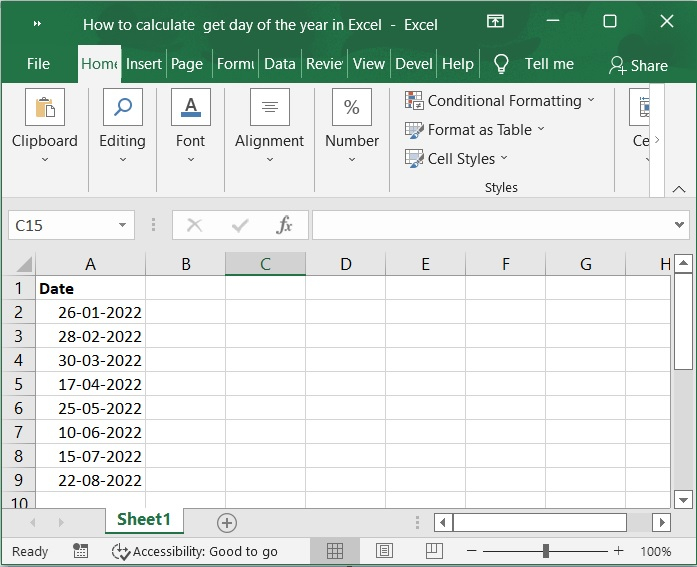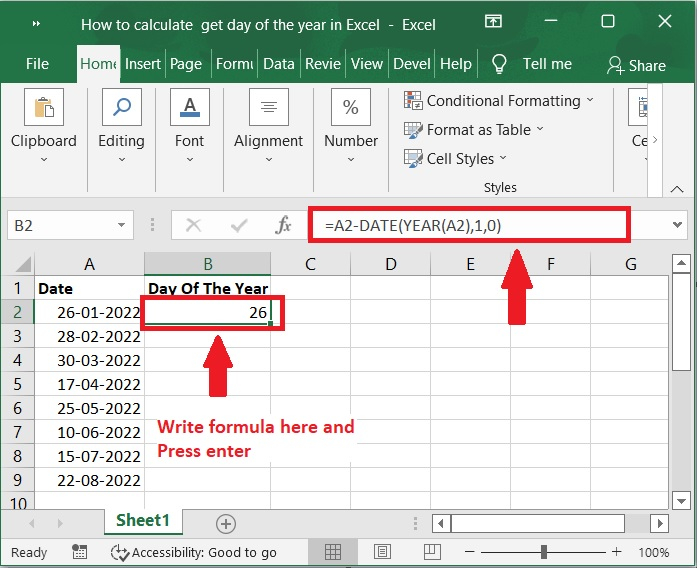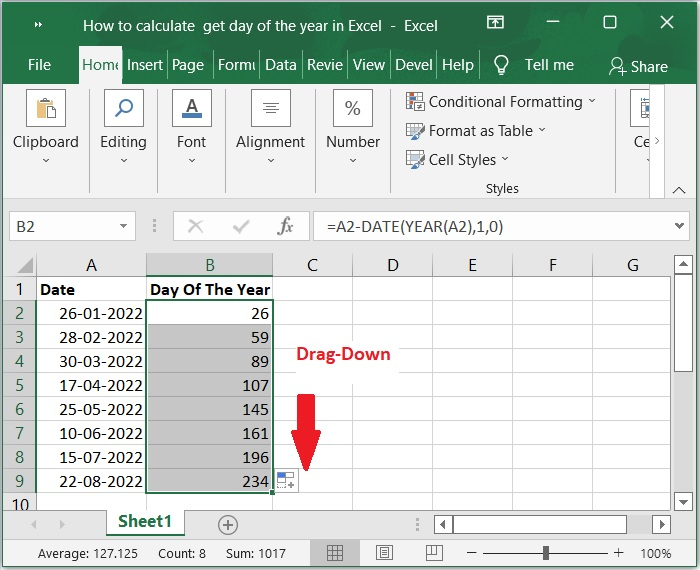# How to calculate / get day of the year in Excel?

Did you ever attempted to determine in Excel which day of the year a particular date falls on based on the 365 days in a year? For instance, the current date is 22-08-2022, and I need to find out which day of the year today is based on the 365 days that make up a year. In this tutorial, I will discuss a straightforward method that may be used to deal with it.

You can solve this problem by using a straightforward formula. We do not have a direct function that allows us to determine the precise day of a year; nevertheless, you can use this formula.

Let’s understand step by step with an example.

## Step 1

In the first step, Let’s assume we have a sample data for calculate or get day of the year. Please refer to the screenshot below.## Step 2

We have a list of dates in cell A2:A10. Now, you want to calculate the day of the year. Then, entered this formula into a blank cell in column B. Please check out the screenshot below for the same.

## Formula

=A2-DATE(YEAR(A2),1,0)Important Note − A2 already contains the date you wish to use to determine the day of the year, but you can modify it as necessary.

## Step 3

Then, the day of the specified date within the year will be displayed when you press the Enter key. Please Check out the screenshot below for the same.The day of the year can also be calculated using the following formula −

=A2-DATE(YEAR(A1),1,1)


## Conclusion

In this tutorial, we used a simple example to help you understand how to calculate or get day of the year by using simple formula in Excel.